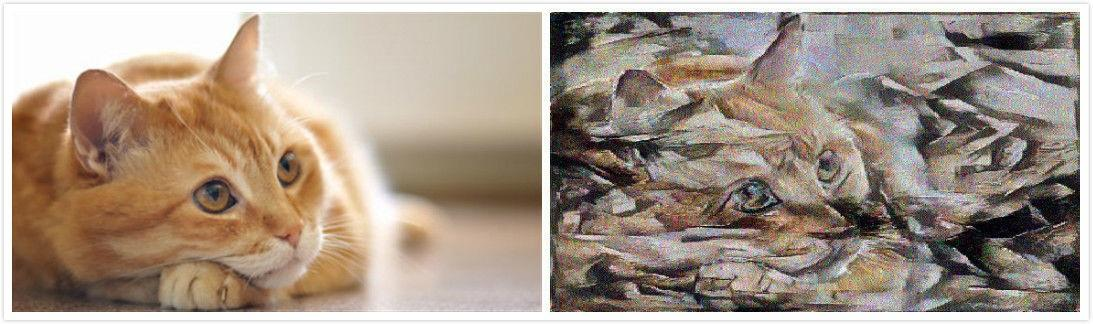“风格迁移”是展示神经网络强大能力的一个很有趣的途径。2015 年，德国和美国的一组研究人员发布了一篇论文《A Neural Algorithm of Artistic Style》

https://arxiv.org/pdf/1508.06576.pdfarxiv.org

walid0925/AI_Artistrygithub.com``````##~~~~~~~~~~~~~~~~~~~~~~~~~~~~~~~~~~~~~~~~~~~~~~~~~~~~~~~~~~~~~~~~~~~~~~~~~~~~##
## Specify paths for 1) content image 2) style image and 3) generated image
##~~~~~~~~~~~~~~~~~~~~~~~~~~~~~~~~~~~~~~~~~~~~~~~~~~~~~~~~~~~~~~~~~~~~~~~~~~~~##

cImPath = './data/base_images/cat.jpg'
sImPath = './data/base_images/violin_and_palette.jpg'
genImOutputPath = './results/output.jpg'

##~~~~~~~~~~~~~~~~~~~~~~~~~~~~~~~~~~~~~~~~~~~~~~~~~~~~~~~~~~~~~~~~~~~~~~~~~~~~##
## Image processing
##~~~~~~~~~~~~~~~~~~~~~~~~~~~~~~~~~~~~~~~~~~~~~~~~~~~~~~~~~~~~~~~~~~~~~~~~~~~~##
from keras import backend as K
from keras.applications.vgg16 import preprocess_input

targetHeight = 512
targetWidth = 512
targetSize = (targetHeight, targetWidth)

cImArr = img_to_array(cImage)
cImArr = K.variable(preprocess_input(np.expand_dims(cImArr, axis=0)), dtype='float32')

sImArr = img_to_array(sImage)
sImArr = K.variable(preprocess_input(np.expand_dims(sImArr, axis=0)), dtype='float32')

gIm0 = np.random.randint(256, size=(targetWidth, targetHeight, 3)).astype('float64')
gIm0 = preprocess_input(np.expand_dims(gIm0, axis=0))
gImPlaceholder = K.placeholder(shape=(1, targetWidth, targetHeight, 3))``````

• F和P是两个矩阵，包含N个行和M个列
• N是给定层L中的过滤器数量，M是给定层I的特征图谱（高度乘以宽度）中空间元素的数量
• F包含给定层L中X的特征表示
• P包含给定层L中p的特征表示

``````def get_feature_reps(x, layer_names, model):
"""
Get feature representations of input x for one or more layers in a given model.
"""
featMatrices = []
for ln in layer_names:
selectedLayer = model.get_layer(ln)
featRaw = selectedLayer.output
featRawShape = K.shape(featRaw).eval(session=tf_session)
N_l = featRawShape[-1]
M_l = featRawShape*featRawShape
featMatrix = K.reshape(featRaw, (M_l, N_l))
featMatrix = K.transpose(featMatrix)
featMatrices.append(featMatrix)
return featMatrices

def get_content_loss(F, P):
cLoss = 0.5*K.sum(K.square(F - P))
return cLoss``````

``````def get_Gram_matrix(F):
G = K.dot(F, K.transpose(F))
return G``````

``````def get_style_loss(ws, Gs, As):
sLoss = K.variable(0.)
for w, G, A in zip(ws, Gs, As):
M_l = K.int_shape(G)
N_l = K.int_shape(G)
G_gram = get_Gram_matrix(G)
A_gram = get_Gram_matrix(A)
sLoss+= w*0.25*K.sum(K.square(G_gram - A_gram))/ (N_l**2 * M_l**2)
return sLoss``````

``````def get_total_loss(gImPlaceholder, alpha=1.0, beta=10000.0):
F = get_feature_reps(gImPlaceholder, layer_names=[cLayerName], model=gModel)
Gs = get_feature_reps(gImPlaceholder, layer_names=sLayerNames, model=gModel)
contentLoss = get_content_loss(F, P)
styleLoss = get_style_loss(ws, Gs, As)
totalLoss = alpha*contentLoss + beta*styleLoss

``````def calculate_loss(gImArr):
"""
Calculate total loss using K.function
"""
if gImArr.shape != (1, targetWidth, targetWidth, 3):
gImArr = gImArr.reshape((1, targetWidth, targetHeight, 3))
loss_fcn = K.function([gModel.input], [get_total_loss(gModel.input)])
return loss_fcn([gImArr]).astype('float64')

"""
Calculate the gradient of the loss function with respect to the generated image
"""
if gImArr.shape != (1, targetWidth, targetHeight, 3):
gImArr = gImArr.reshape((1, targetWidth, targetHeight, 3))

from keras.applications import VGG16
from scipy.optimize import fmin_l_bfgs_b

tf_session = K.get_session()
cModel = VGG16(include_top=False, weights='imagenet', input_tensor=cImArr)
sModel = VGG16(include_top=False, weights='imagenet', input_tensor=sImArr)
gModel = VGG16(include_top=False, weights='imagenet', input_tensor=gImPlaceholder)
cLayerName = 'block4_conv2'
sLayerNames = [
'block1_conv1',
'block2_conv1',
'block3_conv1',
'block4_conv1',
]

P = get_feature_reps(x=cImArr, layer_names=[cLayerName], model=cModel)
As = get_feature_reps(x=sImArr, layer_names=sLayerNames, model=sModel)
ws = np.ones(len(sLayerNames))/float(len(sLayerNames))

iterations = 600
x_val = gIm0.flatten()
xopt, f_val, info= fmin_l_bfgs_b(calculate_loss, x_val, fprime=get_grad,
maxiter=iterations, disp=True)``````

• 尝试用不同的权重：不同的照片混合可能需要调整风格损失权重w或不断优化⍺和 ß的值。例如，在有些例子中，ß/⍺的比例值为10⁵ 效果会更好。
• 尝试用更多的风格层级：这会消耗更多的计算资源，但能够更顺畅地对风格进行迁移。你可以试试VGG19，而不是VGG16，或者将不同的神经网络架构结合在一起。
• 尝试用多张内容照片和风格照片：你可以为损失函数增加几张风格照片，混合多张照片或多种艺术风格。增加内容照片或许会带来更有意思的艺术效果。
• 增加总变分去噪方法：如果你仔细看看上面我得到的照片，你会发现上面有些颗粒状图案——小小的颜色旋涡。用神经网络处理照片通常都会有这个问题，其中一个原因就是照片的有损压缩被带进了特征图谱里。添加总变分去噪可以有效减轻这个问题，点击查看这一步的代码：github.com/llSourcell/A

http://genekogan.com/works/style-transfer/genekogan.com
llSourcell/AI_Artistgithub.com